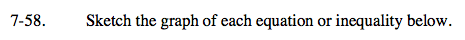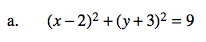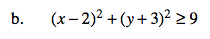Home > A2C > Chapter 7 > Lesson 7.1.4 > Problem7-58

7-58.
1. Sketch the graph of each equation or inequality below. 7-58 HW eTool (Desmos). Homework Help ✎

1. (x − 2)2 + (y + 3)2 = 9

2. (x − 2)2 + (y + 3)2 ≥ 9This equation is already in graphing form. What are the radius and the coordinates of the center?Test a few points to see where the graph should be shaded.

Use the eTool below to sketch the graphs.
Click the link at right for the full version of the eTool: A2C 7-58 HW eTool